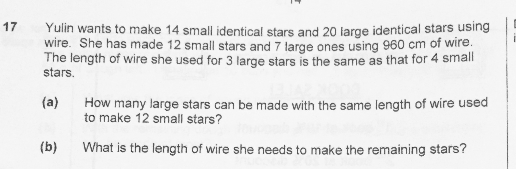# QuestionAnyone help me with attached question

Lenght of wire used to make 4 small stars is the same as 3 large stars

4 small stars  = 3 large stars

Times 3 on both side.

12 small stars = 9 large stars  ( ans for a).

B)

12 small stars + 7 large stars = 960 cm

From ans (a), we know 12 small stars is the same lenght as 9 large stars

9 large stars + 7 large stars = 960cm

16 large stars = 960 cm

1 large stars = 60 cm

4 small stars = 3 large stars

4 small stars = 60 x 3 = 180cm

1 small stars = 180/4 = 45 cm

Total length needed,

14 small stars + 20 large stars.

(14 x 45 )+ (20 x 60) = 1830cm

B)

For the remaining stars, she will need,

1830 – 960 = 870cm #

0 Replies 1 Like ✔Accepted Answer

(a)
12/4 = 3
3 x 3 = 9

(b)
9 + 7 = 16
16 large ——- 960
1 large ——- 960/16 = 60
60 x 3 =180
180/4 = 45 (1 small)
14 – 12 = 2 (remaining small)
20 – 7 = 13 (remaining large)
(2 x 45) + (13 x 60) = 870

Ans : 9 large stars; (b) 870 cm

0 Replies 0 Likes